The congruence condition of triangles is one of the geometry problems we learn in mathematics. We learn under what conditions two triangles can have exactly the same shape.

After learning the triangle congruence theorems, we need to learn how to prove the congruence. You will be asked to prove that two triangles are congruent.

Many people are not good at proofs in math problems. Instead of answering numbers with calculations, you have to prove them with sentences. Since the way to solve them is completely different, many people think that proof problems are difficult.

There are tricks to solving congruence proof problems. We will explain how to solve triangle congruent problems.

## Definition and Symbols of Congruence

What is the definition of congruence in mathematics? Congruence refers to shapes that are exactly the same. Shapes that overlap when flipped over are also congruent.

When shapes are congruent, they are all identical, including the lengths and angles. For example, suppose we have the following congruent figures.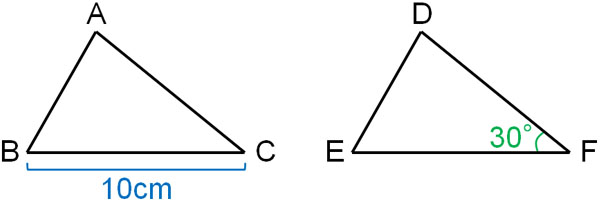Since these two figures are congruent, BC = EF. In other words, the length of side EF is 10 cm. In the same way, ∠C = ∠F. Therefore, the angle of ∠C is 30°. Even if we don’t know the side lengths or angles, we can find the side lengths and angles by using congruence.

In congruence, we use the symbol ≅. In the previous figure, we write △ABC≅△DEF.

When using the symbol for congruence, consider the corresponding points. When two shapes are superimposed, the points in the same part correspond. The corresponding points are shown below.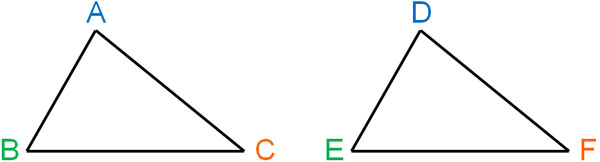When considering the congruence of triangles, the order of the corresponding points must be aligned. For example, for the triangle shown above, the following is correct.

• △ABC≅△DEF
• △ACB≅△DFE

However, if the corresponding points are different, the answer is incorrect. For example, △ABC≅△EFD is incorrect. This is because although the figures are congruent, the corresponding points are different.

### Four Conditions for Triangles to be Congruent

Under what conditions are the two triangles congruent? When learning about congruence in mathematics, it is important to understand the congruence condition. Two triangles are always the same if they satisfy the congruence theorems.

There are four types of congruence theorems for triangles. They are as follows.

• Side – Side – Side (SSS) Congruence Postulate
• Side – Angle – Side (SAS) Congruence Postulate
• Angle – Side – Angle (ASA) Congruence Postulate
• Angle – Angle – Side (AAS) Congruence Postulate

In detail, each of them is as follows.

-Side – Side – Side (SSS) Congruence Postulate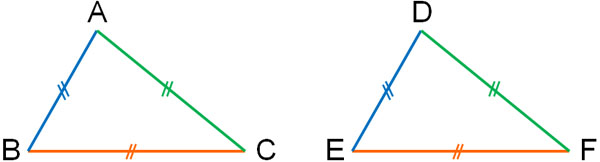If all three sides are equal in length, then the two triangles are congruent.

-Side – Angle – Side (SAS) Congruence Postulate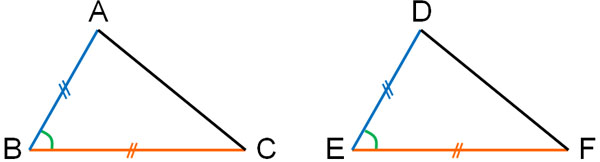Two triangles are congruent if the lengths of the two sides are equal and the angle between the two sides is equal. The angle between the two sides must be equal, and even if the other angles are the same, the triangles are not necessarily congruent.

-Angle – Side – Angle (ASA) Congruence Postulate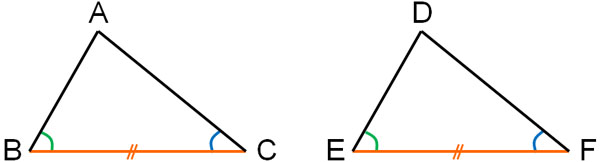Two triangles are congruent if the length of one side is equal and the angles at the ends of the equal sides are the same.

-Angle – Angle – Side (AAS) Congruence Postulate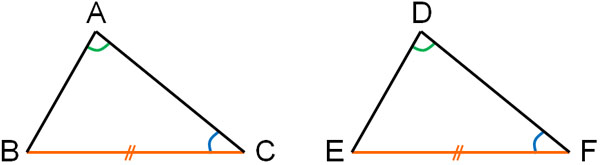Triangles are congruent if the angles of the two pairs are equal and the lengths of the sides that are different from the sides between the two angles are equal.

It is similar to Angle – Side – Angle (ASA) because the two angles are equal. The triangles are congruent even if the equal angles are not the angles at the ends of the sides.

-There Is a Congruence Theorem for Right Triangles

In fact, there are other congruence conditions as well. However, they apply to special triangles. In the case of right triangles, there is another congruence condition. Including right triangles, there are a total of five congruence theorems for triangles.

However, since right triangles are special triangles, we will omit the congruence theorem for right triangles.

### Is It Congruent in the Case of SSA?

In order to prove that triangles are congruent to each other, the triangle congruence theorems must be satisfied. What happens if the congruence condition is not satisfied? In this case, the two triangles are not necessarily congruent. For example, suppose we have two triangles that satisfy the following conditions.

• Side – Side – Angle (SSA)

However, this does not necessarily mean that the triangles are congruent. This is because, for example, we can draw the following triangles.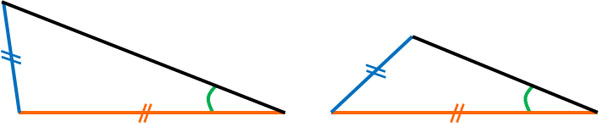The figures satisfy Side – Side – Angle (SSA). However, the two figures are not the same. Therefore, if the two figures do not satisfy the congruence condition of a triangle, they are not congruent.

### Three Types of Congruence Conditions Are Important

When using congruence conditions for triangles, there are three that are particularly important.

• Side – Angle – Side (SAS) Congruence Postulate
• Angle – Side – Angle (ASA) Congruence Postulate
• Angle – Angle – Side (AAS) Congruence Postulate

When proving congruence in mathematics, you will almost always use one of the three theorems. It is possible to prove that triangles are congruent by describing SSS. However, such questions are rarely given.

Of course, it does not mean that there will never be a proof problem of congruence where three sides are equal. However, we often use angles in triangle congruence.

## Prove Using Assumptions and Conclusions

After understanding the triangle congruence theorems, we need to be able to prove that two triangles are congruent. We must be able to solve proof problems.

In order to solve proof problems in mathematics, we need to understand assumptions and conclusions. An assumption is a prerequisite. And by making assumptions, we can often state a conclusion. For example, how about the following case?

• Assumption: $x>5$
• Conclusion: $x>1$

If all numbers are greater than 5, then all numbers are greater than 1. Therefore, if the assumption is $x>5$, we can say that the conclusion ($x>1$) is satisfied. This is the assumption and conclusion.

However, in some cases, the conclusion cannot be stated only by using assumptions. For example, in the following figure where AB=DE and AB||DE, does △ABC≅△EDC hold?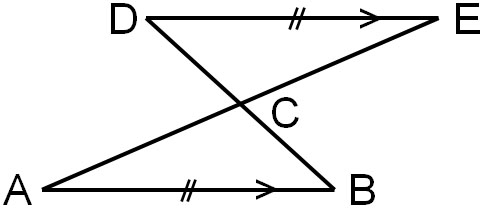In this case, we have the following,

• Assumption: AB=DE and AB||DE
• Conclusion: △ABC≅△EDC

When the sides AB and DE are equal in length and parallel, we cannot understand why △ABC≅△EDC holds. Therefore, when the assumption is true, we need to explain why we can say the conclusion. In mathematics, explaining the reason is called proof.

When it comes to proof, you may think it is difficult. However, it is easy to understand if you realize that it is a rationale for stating a conclusion.

In math calculation problems, we do not know the answer before solving the problem. In a proof problem, on the other hand, the answer (conclusion) is already known. Therefore, try to think of reasons to state the conclusion.

### Steps to Prove Congruence of Triangles

So how do we prove the congruence of triangles? There is a proper procedure to follow when solving proof problems in mathematics. It is as follows.

1. Write down the assumption.
2. Write down your findings with reasons.
3. Use the congruence theorem to prove it.

Let’s check them one by one in detail. Suppose we have the following figure that we noted earlier.If AB=DE and AB||DE, let’s prove △ABC≅△EDC.

-Write Down the Assumption

To prove the congruence of triangles, write the figure you want to prove first. After that, you need to write the assumptions. It is as follows.

• In △ABC and △EDC
• AB=DE: Given – (1).

-Write Down Your Findings with Reasons

Next, describe the reasons to prove that the triangles are congruent. For example, we have the following.

• ∠A = ∠E and AB||DE, the alternate angles in parallel lines are equal – (2).
• ∠B = ∠D and AB||DE, the alternate angles in parallel lines are equal – (3).

The trick to solving triangle proofs is to write down the angles or sides that are equal. For example, in the following cases, we can find out for sure that they are the same.

• Shared lines (overlapping lines): same length.
• The midpoint of the line: the midpoint, so two lines of the same length are created.
• Vertical angles: Same angles.
• Corresponding angles in parallel lines: Same angles.
• Alternate angles in parallel lines: Same angles.

These are just some examples. In any case, by using these properties of shapes, we can find lines of the same length and the same angles.

-Use the Congruence Theorem to Prove It

Finally, state your conclusion based on the assumptions and reasons. It is as follows.

• From (1), (2), and (3), since Angle – Side – Angle (ASA), △ABC≅△EDC

Thus, we have proved that △ABC≅△EDC.

### Pay Attention to the Representation of Angles

In geometry problems, pay attention to the way of representing angles. In geometry problems, we often use three alphabets instead of one to describe the angle.

For example, how would you describe the angle in the following figure?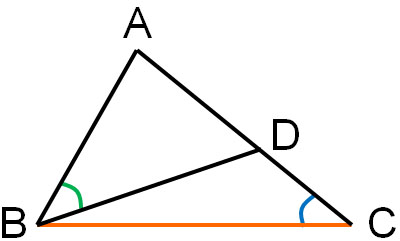∠For angle C, there are no precautions to be taken. On the other hand, what about the angle of B? If you just write ∠B, it is not clear which part of the angle it is. If there are several candidates for the angle, use the three letters of the alphabet.

For example, in the above figure, write ∠ABD. If you use ∠B, it is not clear which angle it is. If you use ∠ABD, it is clear which angle it is.

## Exercise: Proof of Triangles

Q1: Prove the following figure.

For the figure below, △ABC is an equilateral triangle, and when AD=AE and AE||BC, prove that △ABD≅△ACE.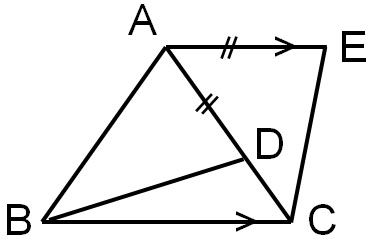• In △ABD≅△ACE
• AD = AE: Given – (1).
• AB = AC: △ABC is an equilateral triangle – (2).
• ∠BAD = ∠CAE: AE||BC, and the alternate angles in parallel lines are equal, so ∠CAE = ∠ACB; also, △ABC is an equilateral triangle, so ∠ACB = ∠BAD – (3).
• From (1), (2), and (3), since Side – Angle – Side (SAS), △ABD≅△ACE

## Learn Congruence Conditions of Triangles and Solve Proof Problems

Triangle proof problems require you to answer in sentences, not in calculations. In proofs, you must remember the triangle congruence theorems. Try to remember all the patterns of when they are congruent.

Then, you will have to prove that they are congruent based on the assumptions. Use the assumptions and describe the facts you have found in order to state the conclusion.

In the proof questions, you already know the answer (conclusion). However, it is unclear which congruence theorem you should use. So use the properties of shapes to find the same sides and angles. If you randomly find common sides and angles, you will be able to satisfy the congruence condition of triangles at some point.

This is the way to prove the congruence of triangles. In proof of figures, the way to solve the problem is different from that of calculation problems. So, let’s understand how to answer them so that you can prove the congruence of triangles.